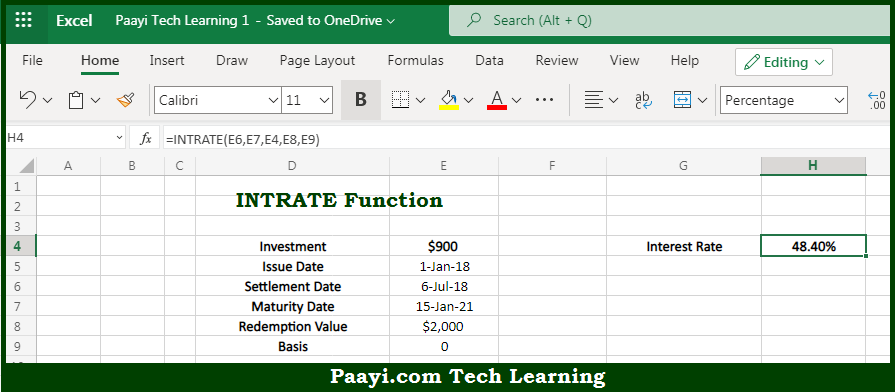# Learn How to Use Microsoft Excel INTRATE Function

Written by | 0 Comments | 548 Views

In this article, you will learn how to use the Microsoft Excel INTRATE function and its prime function in Microsoft Excel. You will also get to know the Microsoft Excel INTRATE function return value and syntax with the help of some examples.

Microsoft Excel INTRATE Function

The main purpose of the Microsoft Excel INTRATE function is to get the interest rate for fully invested security. That implies, with the help of INTRATE function you can able to return the interest rate for a fully invested security. So, with the help of INTRATE function, you can able to get the interest rate for fully invested security.

Return Value of INTRATE Function

The return value will be the interest rate as a percentage.

Syntax of INTRATE Function

=INTRATE(settlement, maturity, investment, redemption, [basis])

Where the arguments:

• settlement: This is the settlement date of the security.
• maturity: This is the maturity date of the security.
• investment: This is the amount originally invested.
• redemption: This is the amount received at maturity.
• basis: This is the day count basis, defaults to 0 (optional).

## How to Use Microsoft Excel INTRATE Function?So we know that with, Microsoft Excel INTRATE function you can able to get the interest rate for fully invested security. That implies, with the help of INTRATE function you can able to return the interest rate for a fully invested security. So, with the help of INTRATE function, you can able to get the interest rate for fully invested security.